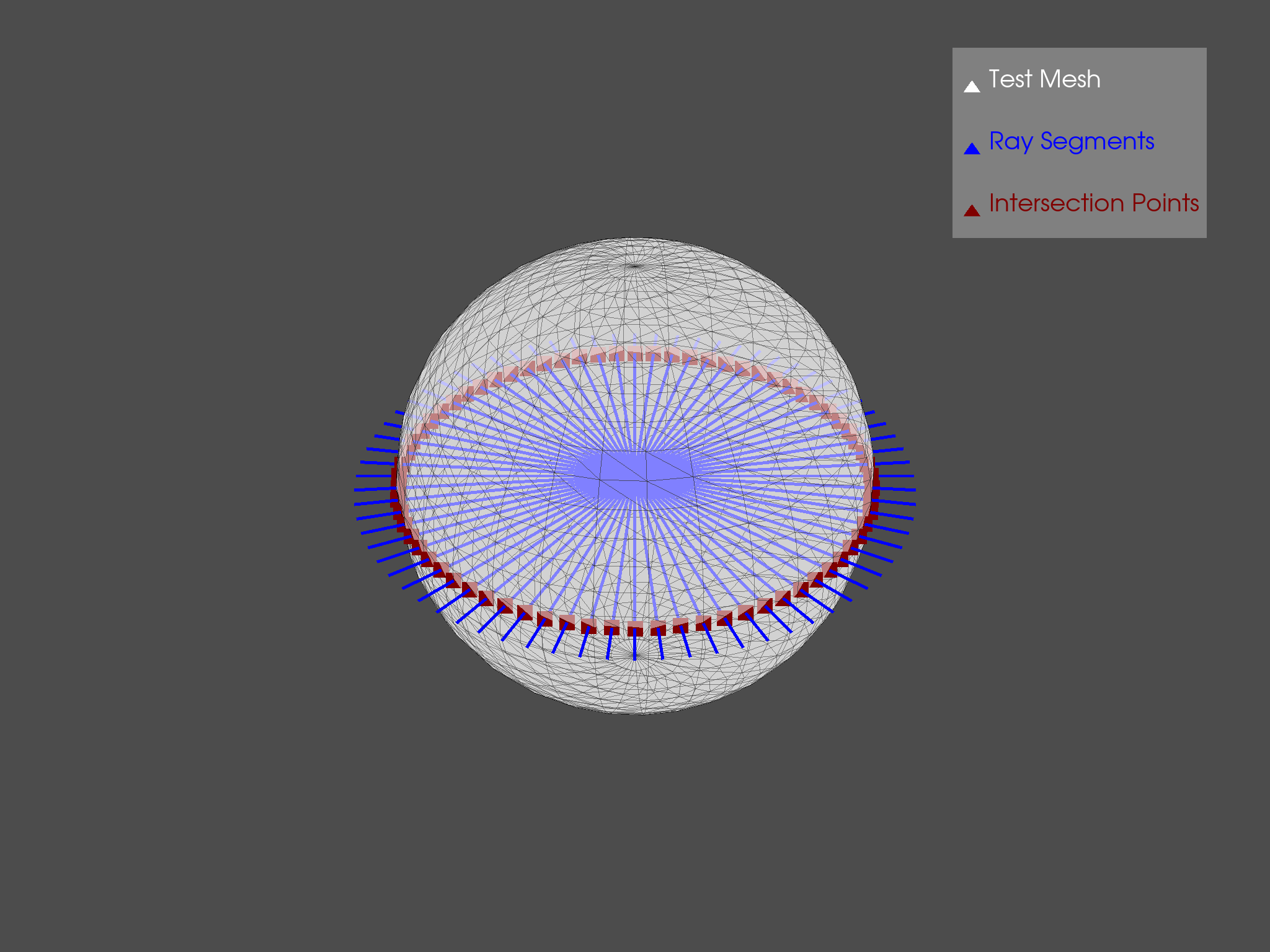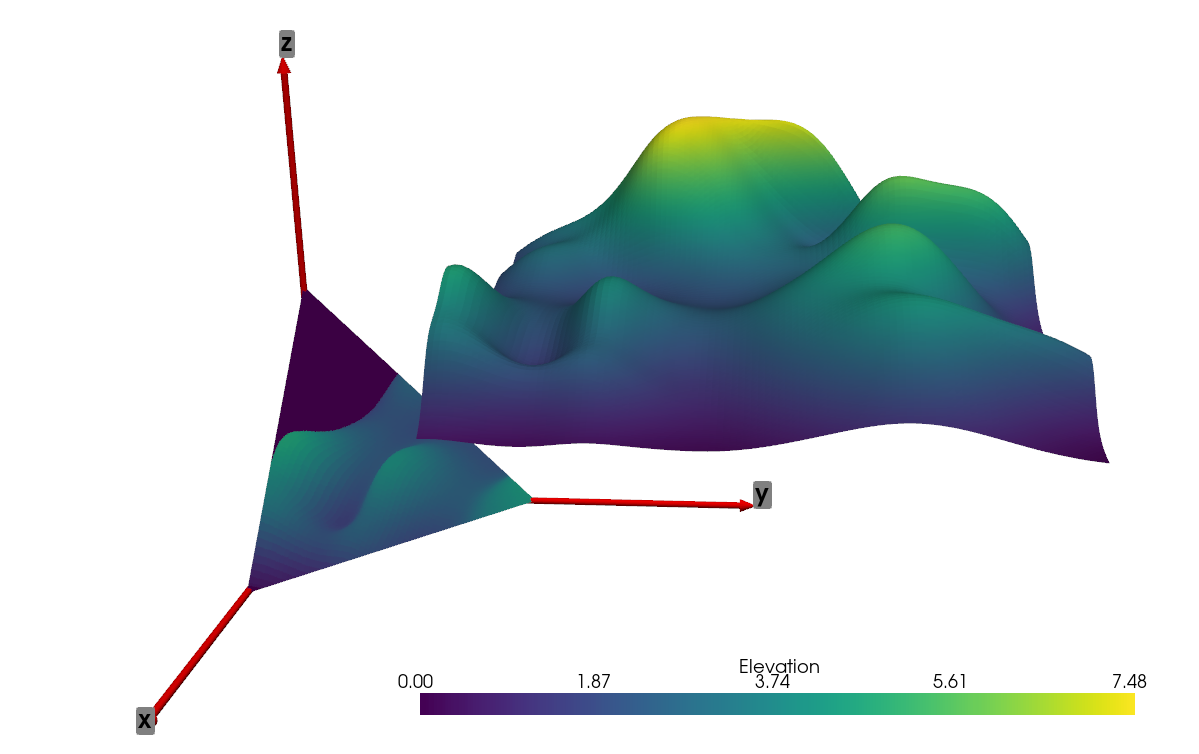# Optional Features#

Due to its usage of numpy, the pyvista module plays well with other modules, including matplotlib, trimesh, rtree, and pyembree. The following examples show some optional features included within pyvista that use or combine several modules to perform advanced analyses not normally included within VTK.

## Vectorised Ray Tracing#

Perform many ray traces simultaneously with a PolyData Object (requires optional dependencies trimesh, rtree and pyembree)

```from math import sin, cos, radians
import pyvista as pv

# Create source to ray trace

# Define a list of origin points and a list of direction vectors for each ray
vectors = [ [cos(radians(x)), sin(radians(x)), 0] for x in range(0, 360, 5)]
origins = [[0, 0, 0]] * len(vectors)

# Perform ray trace
points, ind_ray, ind_tri = sphere.multi_ray_trace(origins, vectors)

# Create geometry to represent ray trace
rays = [pv.Line(o, v) for o, v in zip(origins, vectors)]
intersections = pv.PolyData(points)

# Render the result
p = pv.Plotter()
show_edges=True, opacity=0.5, color="w",
lighting=False, label="Test Mesh")
for ray in rays[1:]:
point_size=25, label="Intersection Points")
p.show()
```## Project to Finite Plane#

The following example expands on the vectorized ray tracing example by projecting the random_hills example data to a triangular plane.

```import numpy as np
from pykdtree.kdtree import KDTree
from tqdm import tqdm

import pyvista as pv
from pyvista import examples

data.translate((10, 10, 10), inplace=True)

# Create triangular plane (vertices [10, 0, 0], [0, 10, 0], [0, 0, 10])
size = 10
vertices = np.array([[size, 0, 0], [0, size, 0], [0, 0, size]])
face = np.array([3, 0, 1, 2])

planes = pv.PolyData(vertices, face)

# Subdivide plane so we have multiple points to project to
planes = planes.subdivide(8)

# Get origins and normals
origins = planes.cell_centers().points
normals = planes.compute_normals(cell_normals=True, point_normals=False)["Normals"]

# Vectorized Ray trace
points, pt_inds, cell_inds = data.multi_ray_trace(
origins, normals
)  # Must have rtree, trimesh, and pyembree installed

# Filter based on distance threshold, if desired (mimics VTK ray_trace behavior)
# threshold = 10  # Some threshold distance
# distances = np.linalg.norm(origins[inds] - points, ord=2, axis=1)
# inds = inds[distances <= threshold]

tree = KDTree(data.points.astype(np.double))
_, data_inds = tree.query(points)

elevations = data.point_data["Elevation"][data_inds]

planes.cell_data["Elevation"] = np.zeros(planes.n_cells)
planes.cell_data["Elevation"][pt_inds] = elevations

# Create axes
axis_length = 20
tip_length = 0.25 / axis_length * 3
tip_radius = 0.1 / axis_length * 3
shaft_radius = 0.05 / axis_length * 3
x_axis = pv.Arrow(
direction=(axis_length, 0, 0),
tip_length=tip_length,
scale="auto",
)
y_axis = pv.Arrow(
direction=(0, axis_length, 0),
tip_length=tip_length,
scale="auto",
)
z_axis = pv.Arrow(
direction=(0, 0, axis_length),
tip_length=tip_length,
scale="auto",
)
x_label = pv.PolyData([axis_length, 0, 0])
y_label = pv.PolyData([0, axis_length, 0])
z_label = pv.PolyData([0, 0, axis_length])
x_label.point_data["label"] = [
"x",
]
y_label.point_data["label"] = [
"y",
]
z_label.point_data["label"] = [
"z",
]

# Plot results
p = pv.Plotter()